• Call Now

1800-102-2727•

# Speed: Definition, Types, Unit

Why is the man walking on the road able to cover less distance in the same time compared to a car that is riding next to him? The reason is that the car travels at a greater speed compared to the man. Similarly, a fast runner covers a greater distance compared to a slow runner because the former has greater speed compared to the latter. Speed is a measure of how fast or how slow someone or something moves.

## What is Speed?

Speed can be defined as the ratio of the distance traveled (d) to the time taken (t). A car moving with a greater speed would be able to reach its destination faster compared to another car that is moving at a slower speed. Mathematically,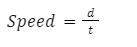Speed is a scalar quantity. The unit of speed is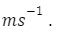## Average Speed

The ratio of total distance traveled by a body to the total time taken by it to cover that distance is called the average speed.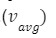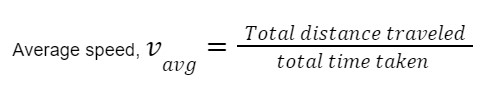A moving body always possesses a positive speed; speed can never be negative.
The slope of the displacement time graph gives aerage speed. Consider the following graph. If “sindicates displacement in time “t”,then between two points a and b,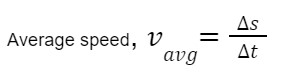## Instantaneous Speed

The instantaneous speed of a particle can be defined as the speed of a particle at a particular moment  of time .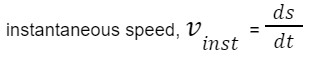i.e first derivative of s wrt time gives instantaneous speed.

Consider the following s-t graph which depicts a non-uniform motion. The tangent drawn to the curve at point P gives the slope at point P, ie. the value of instantaneous speed at that point.## Relative Speed

Let us take for example, three athletes A,B and C who are running a race on the same track, parallel to each other. Now athlete A runs the fastest ,B is faster than C, however slower than A.Fig shows three athletes A, B and C moving at different speeds. B is faster than C but is slower than A

Relative speed gives an idea how one body travels slower or faster in comparison to another. In this case, A moves at a greater speed compared to C, as A moves as compared to B.

The relative speed of A wrt B (when they are moving in the same direction) can be written as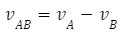Note: When A and B run in opposite directions, relative speed of A wrt B is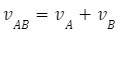## Uniform and Non-uniform Motion

A motion is said to be uniform if the body covers equal distances in equal intervals of time. For eg. if a body covers 2 m of distance each second, the body is said to be in uniform motion. Whereas a motion is said to be non-uniform if the body covers unequal distances in equal intervals of time. A body in non-uniform motion is said to have acceleration.

## Practice Problems of Speed

Question 1. A body travels in a circular path of radius 7 m starting from A to B as shown in figure. The time taken to travel the distance AB is 2 s. What is the average speed of the body?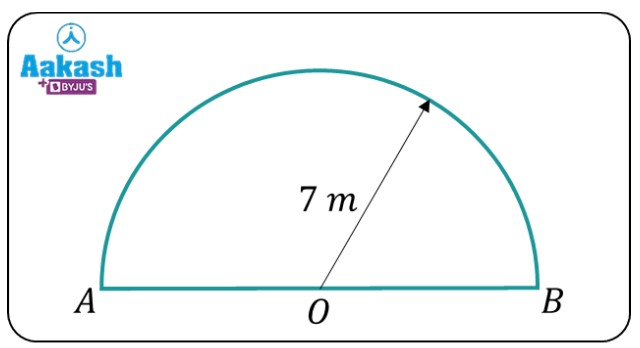(a) 22 m/s (b) 44 m/s (c) 33 m/s (d) 11 m/s

The distance traveled along AB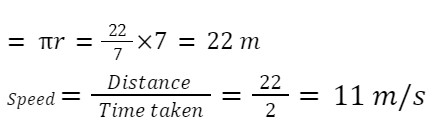Question 2. A car travels with a speed vu from X to Y. It returns from Y to X with a speed vd. What is the average speed of the car during this entire trip?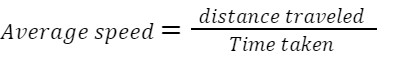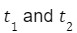be the times taken by the car to go from X to Y and from Y to X .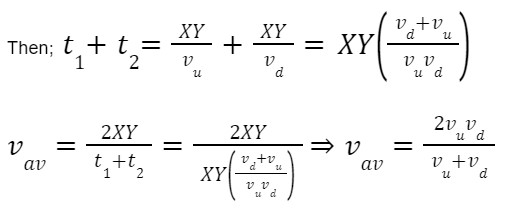Question 3. Person A runs eastwards with a speed of 2 m/s and person B runs westwards with a speed of 1 m/s. What is the relative speed of A wrt B ?

Answer: Since A and B run in opposite directions, relative speed= 2 m/s+1 m/s=3 m/s

Question 4. A car travels a total distance of d. If it travels the first half of the distance with a speed of 8 m/s; with what speed should it travel the second half so that the average speed comes out to be 12 m/s?

(a) 10 m/s (b)12 m/s (c)5 m/s (d)24 m/s

Let the car travel distance d/2 taking time t1 and the next part taking time t2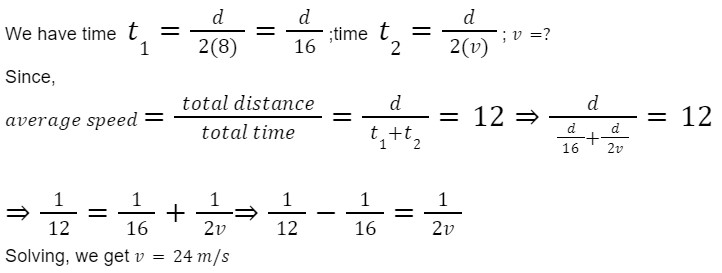## FAQs of Speed

Question 1. What is speed? How is it different from velocity?

Answer: Speed is defined as the ratio of distance to the time taken. Speed is a scalar quantity. Velocity is displacement covered by an object divided by the time taken; and is a vector quantity.

Question 2. What is the unit of speed?

Answer:Distance traveled is the length, so it’s unit is m, and unit of time is s. So, unit of speed is ms-1.

Question 3. Can average velocity be greater than average speed?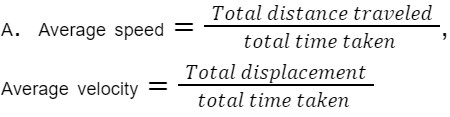Let’s say a body moves from A to B along the semicircle. Now we can clearly see that distance is greater than the magnitude of displacement. Distance = magnitude of displacement for a straight line motion. So, average velocity can never be greater than average speed.

Question 4. Does the speedometer show us average or instantaneous speed?

Answer: The speedometer shows the speed at a given time. So, the speedometer shows us instantaneous speed.